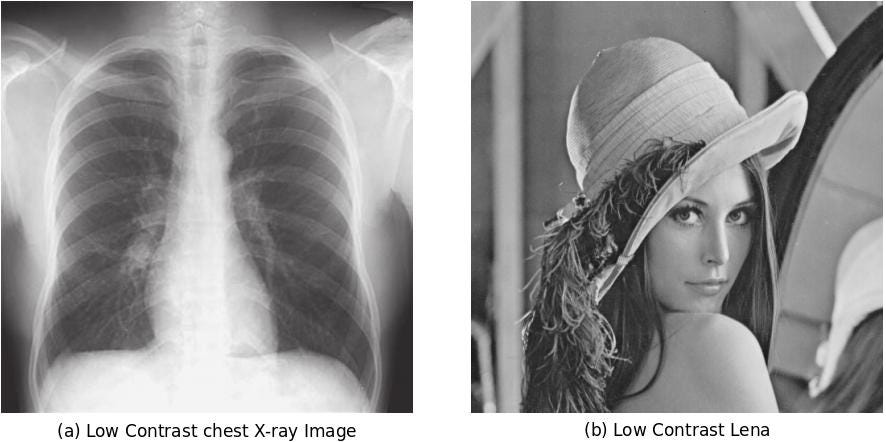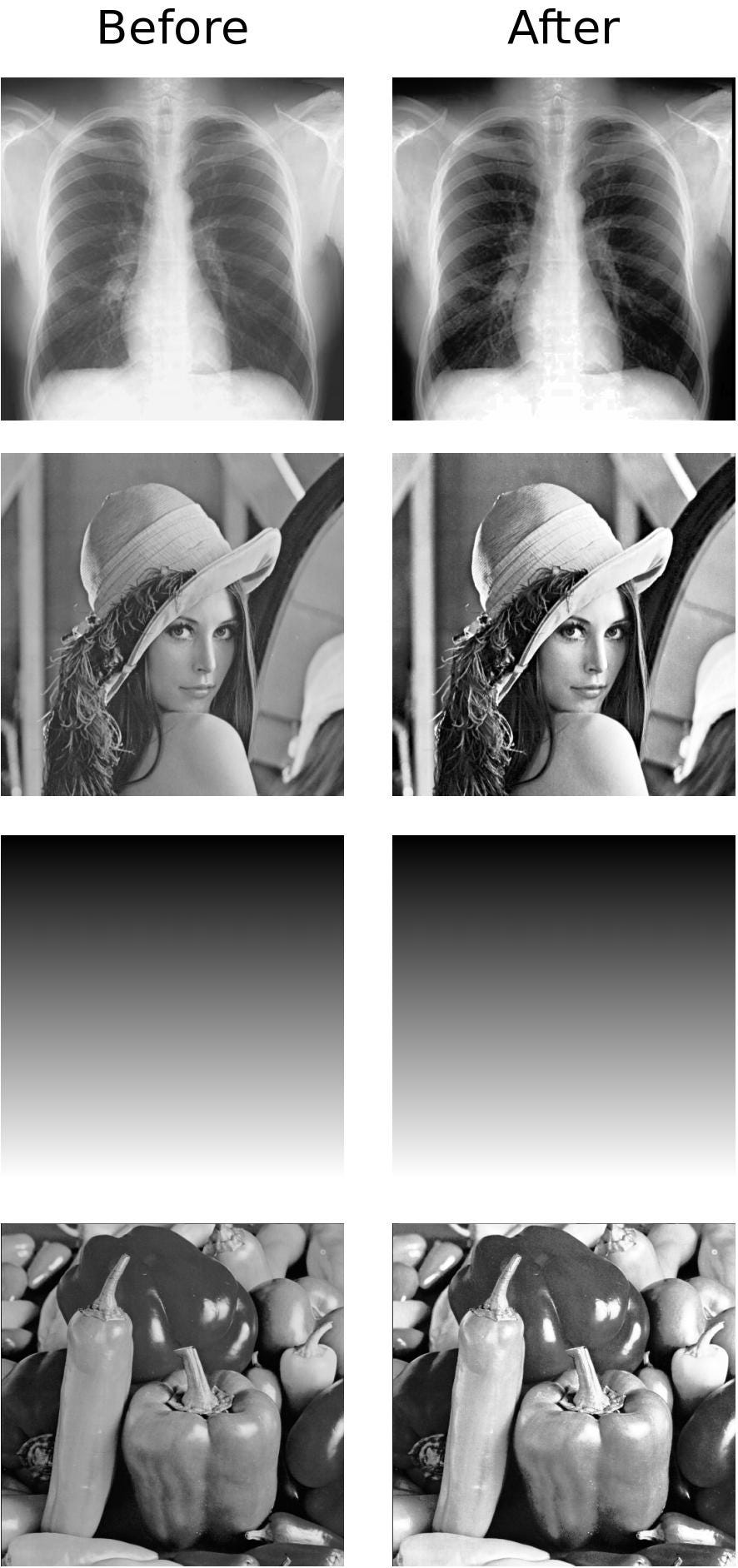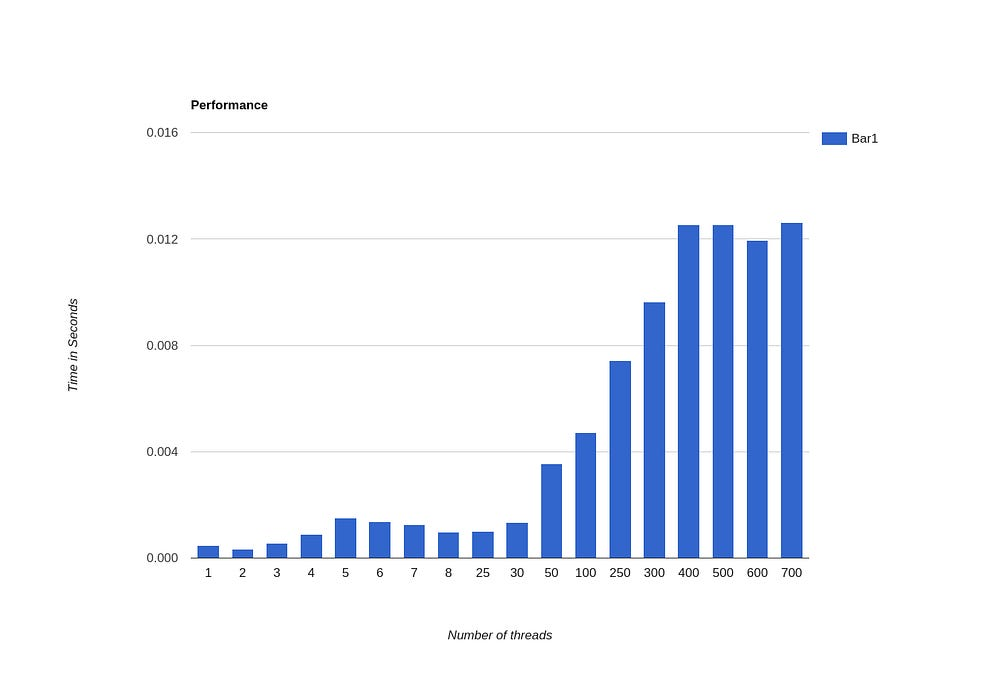# Back-to-basics Part 1: Histogram Equalization in Image Processing

Deep Learning has completely transformed the discipline of Computer Vision and Image Processing entirely. We are quite aware of how Computer Vision has been transformed due to Research around object classification, object detection, object segmentation, human face recognition, human pose estimation and many more. But it was quite astounding for me when I came across the novel works in Image Processing. I would like to mention two very prominent research works here: LSID and ProGANSR do check them out if you haven’t already.

So with all this progress being made in these fields, somewhere I felt that it is more important than ever now to clear the basics of Image Processing in order to gauge the advanced concepts better. So, I am doing this blog series called back-to-basics where I will be reviewing and re-explaining some core concepts of various fields. I will be covering mainly the topics that are somewhat challenging to understand while also being very important for the field.

In this part I will be explaining the concept of Histogram Equalization of Images for improving contrast. The code for this blog is available at my repository -> https://github.com/akanimax/multithreaded-histogram-equalization-cpp. The code is in C++ and uses OpenCV only for reading the images. I am also deviating from my usual Python implementations as this is a Back-to-basics series. So, I thought using C++ is more apt here.

#### Introduction

At times the images captured from various sources lack enough contrast and look washed out. Although they may be bright enough, they still look like something is wrong with them.Examples of Low Contrast Images

Images being bright has to do with the amplitude of their signal while them having low contrast is a fault in the signal’s frequency. The above diagram shows examples of two low contrast images. The on the left is a low contrast Chest X-ray image and on the right is a low contrast version of the famous Lena’s image (This image is used extensively in image processing).

For simplicity, I am considering gray-scale images. but the concept can be applied to RGB colored images as well.

Is there a way to mathematically process these signals and fix them? Well thankfully there are few. First is a very simple technique called Linear Contrast Stretching. But I’wont be explaining it in details here. Just take a look at the formula for performing this stretching:

R(x, y) = (I(x, y) – Imin) * ((Lmax – Lmin) / (Imax – Imin)) + Lmin

I(x, y) => input Image defined as a function of the coordinates

Imax, Imin => maximum and minimum values of the Image

Lmax, Lmin => maximum and minimum signal value range (Typically are 255, 0).

It can be seen how the range of values which the input image has gets stretched to the full range of the signal’s possible values. Note that for specific implementation, we have to round off the R(x, y) values to nearest integers for allowing the hardware to render the image pixel values.

Although the technique is quite useful and simple to implement, it is vulnerable to outlier pixel values. Suppose the image has all the pixels in the range [200 – 255] except one which is completely black i.e has a value of 0, then the contrast stretching won’t work at all.

A more robust technique is the histogram equalization.

#### Histogram Equalization

Histogram is a data-structure to store the frequencies of all the pixel levels in the images. By frequency, I simply mean the number of pixels in the image which have that specific pixel intensity value. The number of bins for the histogram in this case are taken to be equal to the number of pixel intensity levels in the image. Usually, it is 0 – 255.

For equalizing the histogram, we need to compute the histogram and then normalize it into a probability distribution. For normalization, we just need to divide the frequency of each pixel intesity value by the total number of pixels present in the image. This is equal to the resolution of the image. i.e. (Nrows x Ncols). The equalization process makes sure that the resulting histogram is flat. Following is the transformation function for the image in order to obtain a flat histogram.

T(x, y) = (L – 1) * (sum(k, {0 – P(I(x, y))}, Pk(I(x, y))))

sum(k, {a, b}, fk(x)) => summation over f(x) where k goes from a to b.

In order to understand the above formula, we need to know the concept of Cumulative Mass Function (CMF). The CMF of a random variable at a particular value gives the probability that the random variable can take upto the given value. So, basically in the HE formula we are transforming the value of input pixel intensity to such a value which is in sync with CMF of the input image. This is indeed a bit difficult to convey, but you will understand better if you go through the code. This operation ensures that the Histogram of the resulting image is flattened.

#### ImplementationBefore-After examples of Histogram Equalization

Given all the mathematical explanation about the technique, I wrote a C++ implementation for performing this operation. Note that I have used OpenCV only for reading the images. The core logic of histogram equalization doesn’t use any libraries. For improving the performance, I have applied CPU based multi-threading. Given the number of threads as a command line arg, I divide the image into those many vertical bands and give them to multiple threads for processing. Note the histogram calculation is still done sequentially as it needs global information.

The accompanying diagram shows the effect of applying histogram equalization to the low-contrast images. It is really cool to note that no Deep Learning is required here. Just plain and simple math involving signal processing. The technique has been used in numerous image editing applications, but now a days, they use the adaptive DL based image enhancers.

Note how the Example 3 in the accompanying diagram is unaltered by the histogram equalization operation. Since the image has equal number of pixels for every possible value, the histogram is already flat and cannot be equalized further.graph of time taken by the program v/s number of threads

Above graph shows the run-time performance of the application as a function of number of threads spawned to perform the histogram equalization for the same image (number of pixels). You can note that after a certain number of threads, increasing more threads just reduces the performance drastically due to the overhead of creating the threads in the system.

#### Final Comments

This is the first part in the series titled Back-2-Basics in which I’ll be reviewing and explaining some of the basic concepts which are of importance and form a foundation for the higher complicated concepts. Once the series is complete, I’ll create a RI with links to all the articles in this series.

Thank you for reading! Do let me know if you have any suggestions / feedback. Contributions to this series are most welcome!

Source: Deep Learning on Medium